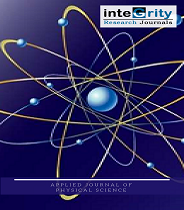# Applied Journal of Physical Science#### APPLIED JOURNAL OF PHYSICAL SCIENCEIntegrity Research Journals

ISSN: 2756-6684
Model: Open Access/Peer Reviewed
DOI: 10.31248/AJPS
Start Year: 2018
Email: ajps@integrityresjournals.org

#### ABSTRACT

In this short paper, we established q- analogues of some well-known inequalities for the classical Gamma function and some inequalities bounding the ratio , where  is the q-analogue of the Gamma function. The method employed in presenting the results made use of strict logarithmic convexity of a function and classical mean value theorem involving the q-analogue of the psi function. At the end, the proven result provided the q-analogue of some known result concerning the classical Gamma function.

#### REFERENCES

 Abramowitz, M., & Stegun, I. (1965). A handbook of Mathematical functions. New York. Andrews, G. E., Askey, R. A., & Roy, R. (1999). Special functions. Cambridge University Press, Cambridge. Crossref Gautschi, W. (1959). Some elementary inequalities relating to the gamma and incomplete gamma function. Journal of Mathematical Physics, 38(1), 77-81. Crossref Hashimu, M., Abdul Razak, Y.A. & Hatsu, E., (2022). Convexity properties and inequalities regarding the q-Gamma function. Applied journal of physical science, 4(4), 37-41. Mansour, T., & Shabani, A. S. (2009). Some inequalities for the q-digamma function. Journal of Inequalities in Pure and Applied Mathematics, 10(1). Link Merkle, M. (2008). Inequalities for the gamma function via convexity. Advances in Inequalities for Special Functions, 81-100. Njionou Sadjang, P. (2017). On two (p, q)-analogues of the Laplace transform. Journal of Difference Equations and Applications, 23(9), 1562-1583. Crossref Qi, F. (1999). Monotonicity results and inequalities for the gamma and incomplete gamma functions. RGMIA Research Report Collection, 2(7). Link Qi, F. (2009). Bounds for the ratio of two Gamma functions-From Wendel's and related inequalities to logarithmically completely monotonic functions. Link Wendel, J. G. (1948). Note on the gamma function. The American Mathematical Monthly, 55(9), 563-564. Crossref